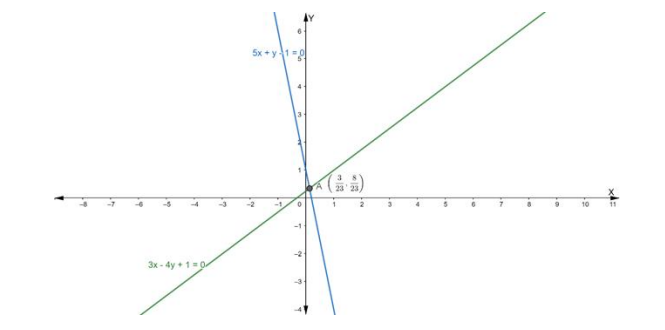# Find the equation of the line passing through the intersection of the linesQuestion:

Find the equation of the line passing through the intersection of the lines $3 x-4 y+1=0$ and $5 x+y-1=0$ and which cuts off equal intercepts from the axes.

Solution:

Suppose the given two lines intersect at a point $P\left(x_{1}, y_{1}\right)$. Then, $\left(x_{1}, y_{1}\right)$ satisfies each of the given equations.

$3 x-4 y+1=0 \ldots$ (i)

$5 x+y-1=0 \ldots$ (ii)

Now, we find the point of intersection of eq. (i) and (ii)

Multiply the eq. (ii) by 4 , we get

$20 x+4 y-4=0 \ldots$ (iii)

On adding eq. (iii) and (i), we get

$20 x+4 y-4+3 x-4 y+1=0$

$\Rightarrow 23 x-3=0$

$\Rightarrow 23 x=3$

$\Rightarrow \mathrm{x}=\frac{3}{23}$

Putting the value of $x$ in eq. (ii), we get

$5\left(\frac{3}{23}\right)+y-1=0$

$\Rightarrow \frac{15}{23}+\mathrm{y}-1=0$

$\Rightarrow \mathrm{y}=1-\frac{15}{23}$

$\Rightarrow \mathrm{y}=\frac{23-15}{23}$

$\Rightarrow \mathrm{y}=\frac{8}{23}$

Hence, the point of intersection $\mathrm{P}\left(\mathrm{x}_{1}, \mathrm{y}_{1}\right)$ is

$\left(\frac{3}{23}, \frac{8}{23}\right)$Now, the equation of line in intercept form is:

$\frac{x}{a}+\frac{y}{b}=1$

where a and b are the intercepts on the axis.

Given that: a = b

$\Rightarrow \frac{x}{a}+\frac{y}{a}=1$

$\Rightarrow \frac{x+y}{a}=1$

$\Rightarrow x+y=a \ldots(i)$

If eq. (i) passes through the point $\left(\frac{3}{23}, \frac{8}{23}\right)$, we get

$\frac{3}{23}+\frac{8}{23}=\mathrm{a}$

$\Rightarrow \frac{11}{23}=\mathrm{a}$

$\Rightarrow \mathrm{a}=\frac{11}{23}$

Putting the value of 'a' in eq. (i), we get

$x+y=\frac{11}{23}$

$\Rightarrow 23 x+23 y=11$

Hence, the required line is 23x + 23y = 11